# Hypergeometric equation

(diff) ← Older revision | Latest revision (diff) | Newer revision → (diff)

Gauss equation

An ordinary second-order linear differential equation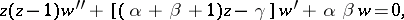(1)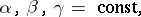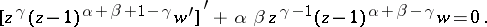The variablesand the parameters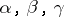assume, in the general case, complex values. After substituting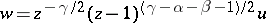the reduced form of equation (1) is obtained: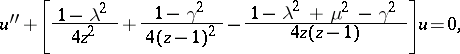(2)

where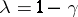,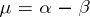, and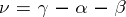.

Equation (1) was studied in detail by C.F. Gauss

in connection with his theory of the hypergeometric series, but had been considered (together with its solution) by L. Euler at an even earlier date.

Solutions of equation (1) are expressed by way of the hypergeometric function. Ifis not an integer, the general solution of (1) may be written as(3)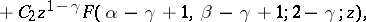where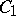and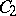are arbitrary constants. The representation (3) is valid in the complex-plane with slits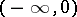and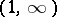. In particular, in the real case (3) yields the general solution of (1) in the interval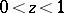. For integral values ofthe general solution is more complicated (the individual terms may contain logarithms).

Functions other than those shown in (3) may also be selected as a fundamental system of solutions of equation (1). For instance, if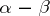is not an integer, then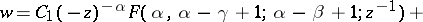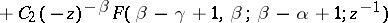is the general solution of (1) in the complex plane with slit, .

The Gauss equations include, as particular cases, a number of differential equations encountered in applications; many ordinary linear second-order differential equations are reduced to (1) by transforming the unknown function and the independent variable . The confluent hypergeometric equation, which is close to equation (1), is particularly important. The ratio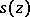of two linearly independent solutions of equation (2) satisfies the Schwarz equation, which is closely connected with the problem of conformal mapping a semi-plane onto a triangle bounded by three peripheral arcs. The study of the inverse function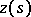leads to the concept of an automorphic function .

There exist linear equations of higher orders whose solutions display properties similar to those of hypergeometric functions: The solution of the following equation of order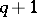,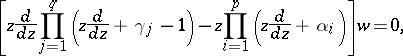is the generalized hypergeometric functionwith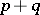parameters. In particular, the generalized hypergeometric equation of the third order, the solution of which is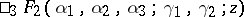, may be represented asHow to Cite This Entry:
Hypergeometric equation. Encyclopedia of Mathematics. URL: http://encyclopediaofmath.org/index.php?title=Hypergeometric_equation&oldid=14414
This article was adapted from an original article by N.Kh. Rozov (originator), which appeared in Encyclopedia of Mathematics - ISBN 1402006098. See original article